# Solving linear equations by graphing#### Everything You Need in One Place

Homework problems? Exam preparation? Trying to grasp a concept or just brushing up the basics? Our extensive help & practice library have got you covered.#### Learn and Practice With Ease

Our proven video lessons ease you through problems quickly, and you get tonnes of friendly practice on questions that trip students up on tests and finals.#### Instant and Unlimited Help

Our personalized learning platform enables you to instantly find the exact walkthrough to your specific type of question. Activate unlimited help now!

0/1
##### Intros
###### Lessons
1. What is interpolation and extrapolation?
0/13
##### Examples
###### Lessons
1. A train travels at a speed of 120km/h. The equation that represents the relationship of distance (d) and time (t) is d=120t.
1. Plot the equation on a graph.
2. Calculate the distance that the train travels in 4.5 hours.
2. The graph shows the relationship of the temperature of water, c, and time, t, as the water boils.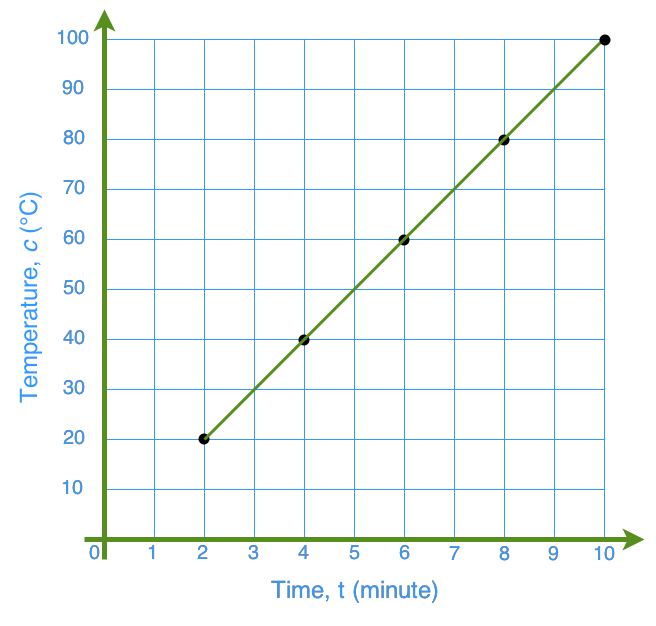1. What is the equation for the relationship?
2. Find the temperature of the water after 7 minutes of boiling.
3. Determine whether it is appropriate to interpolate or extrapolate values on this graph? Why?
3. Plot the graph for each set of values. Then, find the equation that represents each relationship.
1.  x Y -2 -3 -1 0 0 3 1 6 2 9
2.  x Y -2 3 -1 1 0 -1 1 -3 2 -5
3.  x Y -2 -14 -1 -10 0 -6 1 -2 2 2
4.  x Y -2 $- \frac{1}{3}$ -1 $\frac{1}{3}$ 0 $1$ 1 $\frac{5}{3}$ 2 $\frac{7}{3}$
4. Mark ran a marathon. Below is the record of how he did.
 Time, t (h) Distance, d (km) 0.5 4.2 0.8 6.7 1.3 10.9 2.9 24.4 3.5 29.4 4.2 35.3
1. Graph the data above.
2. How far did Mark run in the first 3 hours?
3. Determine the equation that represents the relationship.
4. What was Mark's average speed? Did you make any assumptions?
###### Free to Join!
StudyPug is a learning help platform covering math and science from grade 4 all the way to second year university. Our video tutorials, unlimited practice problems, and step-by-step explanations provide you or your child with all the help you need to master concepts. On top of that, it's fun - with achievements, customizable avatars, and awards to keep you motivated.
• #### Easily See Your ProgressWe track the progress you've made on a topic so you know what you've done. From the course view you can easily see what topics have what and the progress you've made on them. Fill the rings to completely master that section or mouse over the icon to see more details.
• #### Make Use of Our Learning Aids###### Practice Accuracy

Get quick access to the topic you're currently learning.

See how well your practice sessions are going over time.

Stay on track with our daily recommendations.

• #### Earn Achievements as You LearnMake the most of your time as you use StudyPug to help you achieve your goals. Earn fun little badges the more you watch, practice, and use our service.
• #### Create and Customize Your AvatarPlay with our fun little avatar builder to create and customize your own avatar on StudyPug. Choose your face, eye colour, hair colour and style, and background. Unlock more options the more you use StudyPug.
###### Topic Notes
We will learn how to plot linear equations, and then use the graphs as an aid to answer questions about the linear relations. Alternatively, we can find out the linear equations from their graphs. In addition, we will learn how to plot graphs and determine their equations when we are given the table of values of linear relations. We will also explore the concepts of interpolating and extrapolating data from graphs of linear equations.

## How to solve an equation by graphing

A linear equation gives us a straight line when we graph out the points on it. They have a linear relationship. So how do we go about solving an equation by graphing? In this lesson, we'll specifically be focusing on linear equations.

## How to graph linear equations

The first thing you'll have to do in order to graph a linear equation is to put the equation into the slope intercept form. When writing linear equations, you can write it a variety of ways. The slope intercept form looks like this:

y = mx + b

You may need to rearrange some of the terms around to help you isolate y to the left side of the equation before you can get it to look like the slope intercept form equation. Once you are done this, you can move on to step 2.

For this second step, plot out the y-intercept of the equation. Find out what y's coordinate is when x is equalled to 0. This will give you the y-intercept. At times, you may find that your equation doesn't give you a y-intercept (which is times when there is no "b" in your slope intercept form equation). In that case, your first point will be at 0,0 instead of at the y-intercept.

Thirdly, we'll find the second point that we'll be noting down. This will be found through the slope. When you have an equation in the form of a slope-intercept form, the "m" is your slope. The slope m is equalled to rise/run: the rise is how far it goes on the y-axis and the run is how far it goes on the x-axis. Using this knowledge, let it help you find where the second point is based off your first point. So for example, in step 2, if you found that a point was at (0, -2) and your "m" was 1, then your $\frac{rise}{run}$ is equalled to $\frac{1}{1}$. This means you'd move 1 point up on the y-axis, and 1 point to the right on the x-axis. You'll end up with the second point being at (1,-1).

For the fourth and last step, all you'll have to do is draw a line between the two points you've found from the above steps. Make sure that your graph is point the right way depending on if the slope is positive, negative, 0 or undefined.

## Example problems

It's time to put the steps we just learned into use and see how to graph equations. Here are some example problems for you to walk through.

Question 1:

A train travels at a speed of 120km/h. The equation that represents the relationship of distance (d) and time (t) is d=120t.

Plot the equation on a graph

Solution:

The equation in this question is d=120t, which resembles y=mx+b. This means our equation is in slope intercept form.

So, draw the x and y axis

120 is the slope. Slope $\frac{rise}{run} = \frac{120}{1}$

Since it is 120km/hour, it means the train moves 120km every hour. So the graph will look like this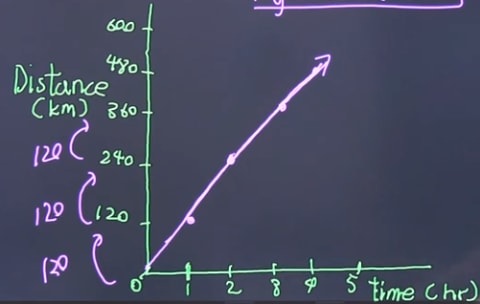Graph of the speed of a train

Question 2:

A train travels at a speed of 120km/h. The equation that represents the relationship of distance (d) and time (t) is d=120t.

Calculate the distance that the train travels in 4.5 hours.

Solution:

Plug the number into the equation

d = 120t

d = 120(4.5)

= 540 km

Question 3:

The graph shows the relationship of the temperature of water, c, and time, t, as the water boils.

What is the equation for the relationship?

Solution:

Try to write the equation in slope intercept form y = mx + b

So, look for the slope from the graph: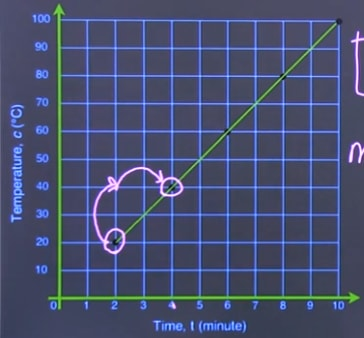Find the slop of the graph

m = slope = $\frac{rise}{run} = \frac{20}{2} = 10$

So the equation is: c = 10t

Question 4:

The graph shows the relationship of the temperature of water, c, and time, t, as the water boils.

Find the temperature of the water after 7 minutes of boiling.

Solution:

From the graph, we know that at 7 minutes the temperature is 70°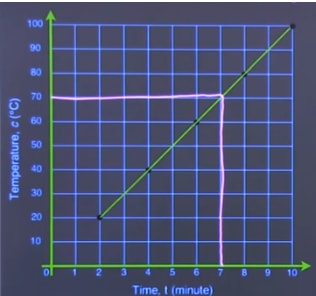Find the temperature after 7 minutes

We can also get the answer algebraically

c = 10t

c = 10(t)

c = 70°C

Question 5

Plot the graph for each set of values. Then, find the equation that represents each relationship.

 x Y -2 -3 -1 0 0 3 1 6 2 9

Solution:

Draw the appropriate x and y axis from the above table of values. This is a method to graphing linear equations.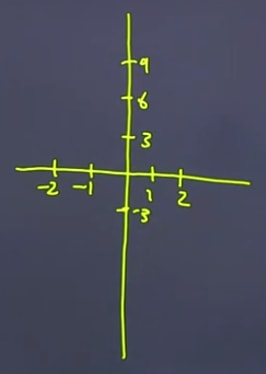Draw x and y axis according to the data table

Plot the point based on the table, and draw the line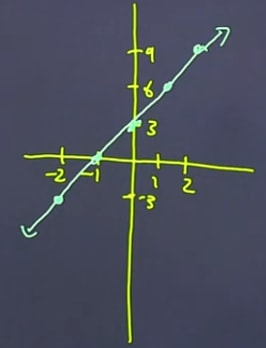Plot the points and draw the line

Now, let's determine the equation.

It will be in this form: y = mx + b. We need to know the "m" and the "b", where m = slope. To find this we can calculate using the slope equation $\frac{y_{2} - y_{1}}{x_{2} - x_{1}}$.

slope = $\frac{y_{2} - y_{1}}{x_{2} - x_{1}} = \frac{6 - 3}{1 - 0} = \frac{3}{1} = 3$